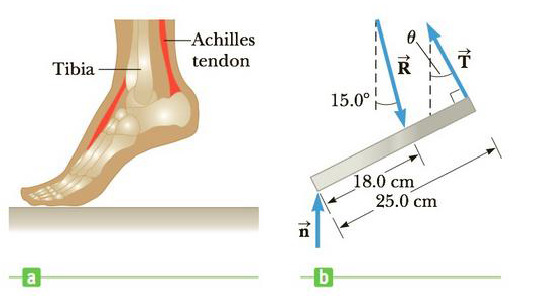Chapter 8, Problem 24P

Chapter
Section
Textbook Problem

When a person stands on tiptoe (a strenuous position), the position of the foot is as shown in Figure P8.24a. The total gravitational force on the body, F → g , is supported by the force n → exerted by the floor on the toes of one foot. A mechanical model of the situation is shown in Figure P8.24b, where T → is the force exerted by the Achilles tendon on the foot and R → is the force exerted by the tibia on the foot. Find the values of T, R, and θ when Fg = n = 700. N.Figure P8.24

To determine
The values of T, R and θ .

Explanation

Given Info: The total gravitational force on the body is Fg , force exerted by the floor on the toes is n, force exerted by the Achilles tendon on the foot is T and force exerted by the tibia on the foot is R.

Explanation:

The following free body diagram shows the force acts on the foot.

From the free body diagram, employing equilibrium condition at the point O (pivot point) in the horizontal position is,

Fx=0

• Fx is the net force acting on the foot

Write the formula to calculate Fx .

Fx=Rsin15οTsinθ

Rewrite the equilibrium condition by substituting the above relation for Fx .

Rsin15οTsinθ=0

The above relation gives the following result.

Rsin15ο=Tsinθ (1)

• Fx is the force acts in the horizontal direction.
• R is the force exerted by the tibia on the foot.
• T is the force exerted by the Achilles tendon on the foot.

Rewrite the above relation in terms of R.

R=Tsinθsin15° (2)

From the free body diagram, employing equilibrium condition at the point O (pivot point) in the vertical position is,

Fy=0700NRcos15°+Tcosθ=0

Rewrite the above relation.

(700N)+Tcosθ=Rcos15ο (3)

At the point O, the net torque is zero. Therefore,

τO=0(700N)(18.0cm)cosθ+T(25.0cm18.0cm)=0 . (4)

From the above equations,

(1+tan215ο)cos4θ+[(2tan15ο)(0

Still sussing out bartleby?

Check out a sample textbook solution.

See a sample solution

The Solution to Your Study Problems

Bartleby provides explanations to thousands of textbook problems written by our experts, many with advanced degrees!

Get Started